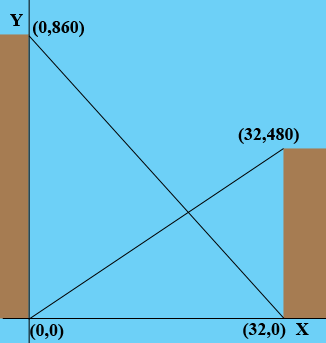SEARCH HOMEMath Central Quandaries & QueriesQuestion from Bill: I need to find the height of the intersection point of two building wires Building A is 860 feet high and building B is 480 feet high. The wires existent from the top of one building to The bottom of the other The distance between is 32 feetHi Bill,

I drew a diagram and put a coordinate system on it with the origin at the base of the taller building.Using the diagram what is the equation of the wire (straight line) from the base of the taller building to the top of the shorter building? What is the equation of the line from the top of the taller building to the base of the shorter building? What is the Y-coordinate of the point where these lines intersect?

PennyMath Central is supported by the University of Regina and The Pacific Institute for the Mathematical Sciences.